# Quantitative Aptitude Quiz for Prelims Exams- SBI & IBPS 2020- 16th December

Q1. The salary of Sumit is Rs. 50000 per month from which he spends 20% on rent, 10% of remaining on bills, 20% of further remaining on miscellaneous expenses. From the remaining amount, he gives some money to his wife and rest he invests in mutual funds in the ratio of 5 : 4. Amount spent on rent is how much less/more than amount invested in mutual funds.
(a) 2800 Rs.
(b) 2400 Rs.
(c) 2600 Rs.
(d) 3200 Rs.
(e) 3000 Rs.

Q2. Due to new rules of TRAI, Rs. 154 is fixed as FTA upto 100 SD channels and additional Rs. 10 per 3 HD or 6 SD channels. A person paying Rs. 400 earlier, has now subscribed for 80 SD channel pack worth Rs. 250 and 9 HD channels pack at Rs. 4 per channel. What is his percent increase in bill amount?
(a) 32.5%
(b) 13.25%
(c) 20%
(d) 17.5%
(e) None of these

Q3. In a company, the ratio of female technical to female non-technical staff is 5:4. There are 64% males in the company. Females in technical are half of males in technical. What is the ratio of male technical staff to that males non technical staff?
(a) 2:1
(b) 3:5
(c) 5:3
(d) 5:4
(e)Cannot be determined

Q4. When digits of the two digits number are reversed, number obtained is 9 less than twice of the original number. Also the new number obtained is 175% of the original number. Find the sum of the digits of the number?
(a) 13
(b) 10
(c) 9
(d) 12
(e) 15

Q5. There are 40 boys in a class A and 60 girls in class B. if girls in class A are 80% of girls in class B. total students in class B are 50% more than total students in class A. find number of boys in class B. (total students = no. of boys + no. of girls)
(a) 72
(b) 75
(c) 80
(d) 62
(e) 78

Q6. Varun and Kartik purchased the shares in ratio 7:9 for the cost of their basic salaries. The company gave each of them 50 shares as incentive, due to which the ratio changes to 9:11. If each share cost is Rs. 60. Find basic salary of Varun.
(a) Rs. 13500
(b) Rs. 16500
(c) Rs. 21000
(d) Rs. 10500
(e) Rs. 27000

Q7. The ratio of first class fare to second class fare is 3:1. No. of tickets booked of first class to second class is in ratio 2:3. Total fare collected was Rs. 1800. Find fare collected from passengers of second class.
(a) Rs. 1200
(b) Rs. 600
(c) Rs. 900
(d) Rs. 750
(e) Rs. 450

Q8. A pizza is cut into two pieces in the ratio of 3 : 7 by weight .The bigger of the two pieces is further cut in the ratio of 4 :7 by weight. Find the ratio of each of the three pieces.
(a) 11 : 14 : 7
(b) 33 : 28 : 49
(c) 35 : 49 : 40
(d) 14 : 19 : 23
(e)none of these

Q9. Rohit scores 84 in Maths while 79 in Science. Karan scores 85 in Maths. If average score of Karan is 6 more than average score of Rohit in both the subjects. Find the marks scored by Karan in Science.
(a) 90
(b) 85
(c) 87.5
(d) 81.5
(e) 85.5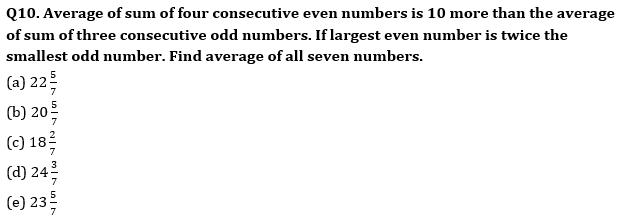Q11. Average of 8 consecutive odd numbers is 10. What will be the average of smallest 4 numbers out of 8 numbers?
(a) 7
(b) 8
(c) 6
(d) 4
(e) 5

Q12.The average weight of 7 students in a class is 26.5 kg. When a new student joins them, the average weight increases by 2.5 kg.Find the weight of the new student?(in kg)
(a) 46.5
(b) 43.5
(c) 48.5
(d) 39.5
(e) 41.5

Q13. Sum of A’s and B’s age 6 years ago is 88. A’s age 18 yrs ago is equal to B’s age 6 years ago. Find the age of A two year hence?
(a) 58 yrs
(b) 64 yrs
(c) 42 yrs
(d) 52 yrs
(e) 48 yrs

Q14. Ratio of age of Ravi to Vicky, 4 years ago was 5: 6, while ratio of present age of Rocky to that of Vicky is 5: 4. If 2 years later sum of age of Ravi and Rocky will be 63 years, then find the difference between present age of Ravi and Vicky?
(a) 4 years
(b) 2 years
(c) 8 years
(d) 6 years
(e) 5 years

Q15. Sum of ages of A and B is 12 years more than twice the age of C and Sum of ages of A & D is twice the age of C. If the average age of B&D is 50 years and average age of all four is also 50 years, then find the difference between the age of A and C?
(a) 6 years
(b) 1 years
(c) 3 years
(d) 4 years
(e) 2 years

Practice More Questions of Quantitative Aptitude for Competitive Exams:

###### Prelims Quiz Study Plan For SBI & IBPS Exams 2020

Solutions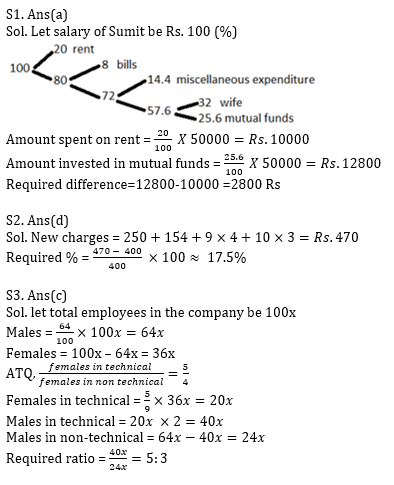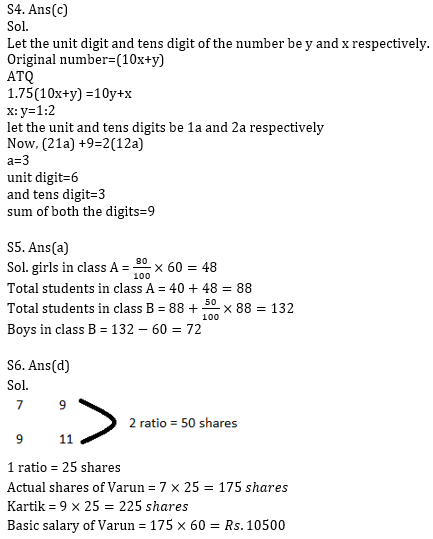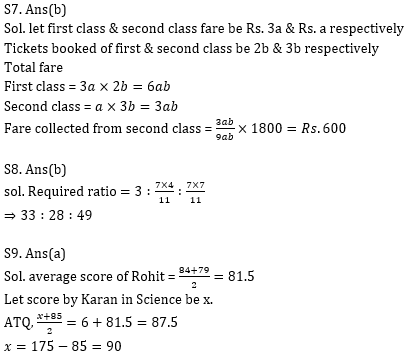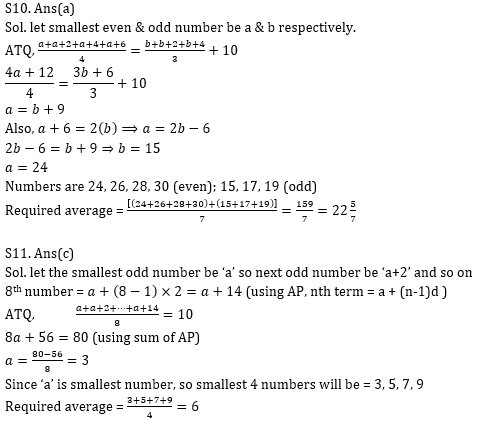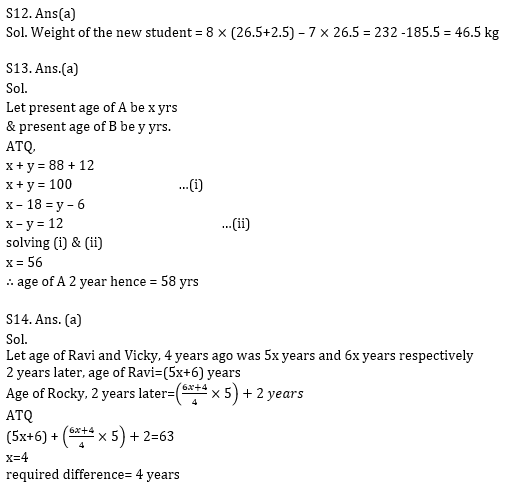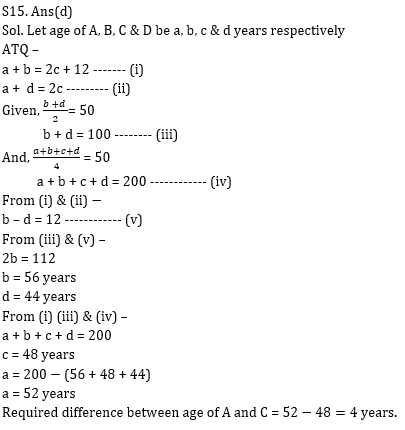Practice with Crash Course and Online Test Series for IBPS Clerk Prelims: